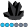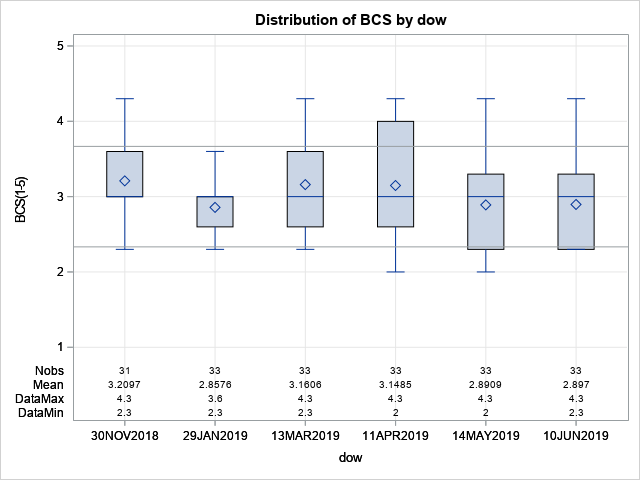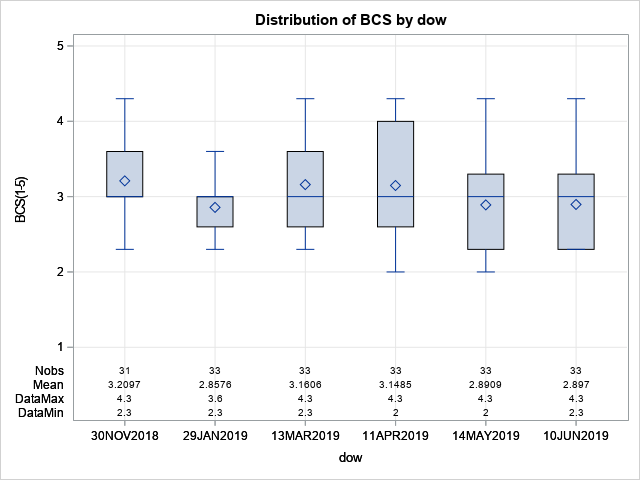das
Obsidian | Level 7

## Adding a reference band to proc boxplot graph

I am wondering how best to add a reference 'band' to a proc boxplot graph.

I have been able to add reflines (vref=) at the lower and upper limits but cannot change color (cvref) while using ods graphics. Here is the code and output.

``````proc boxplot data=work.living ;
by housing ;
where dow>'01NOV2018'd and age_today>=6 and housing="CF-2";
plot bcs*dow /
boxstyle=schematicid
vaxis=1 to 5 by 1
grid
vref=2.333 3.667
;
id db108;
insetgroup min max mean n ;
run;``````But I'd rather use a lightly colored (transparent) band that indicates the reference range. I think I should be able to do this using an annotation data set (annoref) that defines a rectangle. But I seem to get nothing on the graph and nothing in the log to indicate why. Here is my current code, resulting graph and log for everything.

``````data work.annoref;
retain	drawspace "WALLPERCENT" ;
length function \$9 ;
input function \$ height width x1 y1 ;
datalines;
rectangle	10	70	10	50
;
run;

proc print data=work.annoref ; run;

proc boxplot data=work.living ;
by housing ;
where dow>'01NOV2018'd and age_today>=6 and housing="CF-2";
plot bcs*dow /
boxstyle=schematicid
vaxis=1 to 5 by 1
grid
annotate=work.annoref
;
id db108;
insetgroup min max mean n ;
run;````````````791  title; title2; footnote;
792  proc boxplot data=work.living ;
793      by housing ;
794      where dow>'01NOV2018'd and age_today>=6 and housing="CF-2";
795      plot bcs*dow /
796              boxstyle=schematicid
797              vaxis=1 to 5 by 1
798              grid
799              vref=2.333 3.667
800          ;
801      id db108;
802      insetgroup min max mean n ;
803  run;

NOTE: Writing HTML Body file: sashtml2.htm
NOTE: Processing beginning for PLOT statement number 1.
NOTE: Processing started for the BY group housing=CF-2.
NOTE: Since the format DATE is associated with the group variable dow, it is assumed that the
natural interval between group positions is one DAY.
NOTE: The REPEAT option is assumed when a DATE format is used with a group variable.
NOTE: The above message was for the following BY group:
housing=CF-2
NOTE: There were 196 observations read from the data set WORK.LIVING.
WHERE (dow>'01NOV2018'D) and (age_today>=6) and (housing='CF-2');
NOTE: PROCEDURE BOXPLOT used (Total process time):
real time           1.34 seconds
cpu time            0.18 seconds

804
805  data work.annoref;
806  retain  drawspace "WALLPERCENT" ;
807  length function \$9 ;
808  input function \$ height width x1 y1 ;
809  datalines;

NOTE: The data set WORK.ANNOREF has 1 observations and 6 variables.
NOTE: DATA statement used (Total process time):
real time           0.03 seconds
cpu time            0.03 seconds

811  ;
812  run;
813
814  proc print data=work.annoref ; run;

NOTE: There were 1 observations read from the data set WORK.ANNOREF.
NOTE: PROCEDURE PRINT used (Total process time):
real time           0.23 seconds
cpu time            0.03 seconds

815
816  proc boxplot data=work.living ;
817      by housing ;
818      where dow>'01NOV2018'd and age_today>=6 and housing="CF-2";
819      plot bcs*dow /
820              boxstyle=schematicid
821              vaxis=1 to 5 by 1
822              grid
823              annotate=work.annoref
824          ;
825      id db108;
826      insetgroup min max mean n ;
827  run;

NOTE: Processing beginning for PLOT statement number 1.
NOTE: Processing started for the BY group housing=CF-2.
NOTE: Since the format DATE is associated with the group variable dow, it is assumed that the
natural interval between group positions is one DAY.
NOTE: The REPEAT option is assumed when a DATE format is used with a group variable.
NOTE: The above message was for the following BY group:
housing=CF-2
NOTE: There were 196 observations read from the data set WORK.LIVING.
WHERE (dow>'01NOV2018'D) and (age_today>=6) and (housing='CF-2');
NOTE: PROCEDURE BOXPLOT used (Total process time):
real time           0.62 seconds
cpu time            0.10 seconds

``````

I know I'm going to have to set the rectangle dimensions better, but I wanted just to see something before I tried to figure that part out next.

Thank you,

Dave

1 ACCEPTED SOLUTION

Accepted SolutionsDanH_sas
SAS Super FREQ

## Re: Adding a reference band to proc boxplot graph

I'm not sure of your SAS version, but this is something you can do in SGPLOT:

``````proc sgplot data=sashelp.heart;
band x=sex upper=230 lower=180 / name="conf" legendlabel="confidence region";
vbox cholesterol / category=sex displaystats=(min max mean n);
keylegend "conf";
run;``````

Give that a try and see if it works for you.

Thanks!
Dan

2 REPLIES 2DanH_sas
SAS Super FREQ

## Re: Adding a reference band to proc boxplot graph

I'm not sure of your SAS version, but this is something you can do in SGPLOT:

``````proc sgplot data=sashelp.heart;
band x=sex upper=230 lower=180 / name="conf" legendlabel="confidence region";
vbox cholesterol / category=sex displaystats=(min max mean n);
keylegend "conf";
run;``````

Give that a try and see if it works for you.

Thanks!
Dandas
Obsidian | Level 7

## Re: Adding a reference band to proc boxplot graph

Thank you DanH_sas. That works nicely in 9.4. Here is my code and example figure. I like it a lot.

``````proc sgplot data=work.living ;
by housing ;
where dow>'01NOV2018'd and age_today>=6;
band x=dow upper=3.667 lower=2.333 / name="nr" legendlabel="normal range";
vbox bcs / category=dow displaystats=(min max mean n);
xaxis type=time display=(nolabel);
yaxis values=(1 to 5 by 1) grid ;
keylegend "nr";
run;``````Discussion stats
• 2 replies
• 763 views
• 2 likes
• 2 in conversation